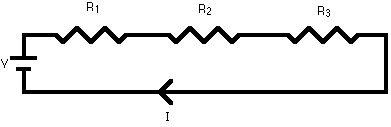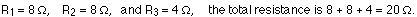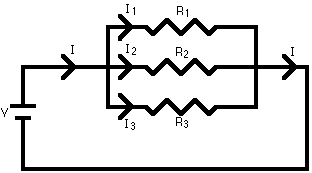## Friday, 3 October 2014

### SERIES AND PARALLEL COMBINATION OF RESISTORS

EXAMPLE 1
Equivalent resistance of resistors in series : R = R1 + R2 + R3 + ...A series circuit is shown in the diagram above. The current flows through each resistor in turn. If the values of the three resistors are:With a 10 V battery, by V = I R the total current in the circuit is:
I = V / R = 10 / 20 = 0.5 A. The current through each resistor would be 0.5 A.

EXAMPLE 2

Equivalent resistance of resistors in parallel: 1 / R = 1 / R1 + 1 / R2 + 1 / R3 +...A parallel circuit is shown in the diagram above. In this case the current supplied by the battery splits up, and the amount going through each resistor depends on the resistance. If the values of the three resistors are:With a 10 V battery, by V = I R the total current in the circuit is: I = V / R = 10 / 2 = 5 A.
The individual currents can also be found using I = V / R. The voltage across each resistor is 10 V, so:
I1 = 10 / 8 = 1.25 A
I2 = 10 / 8 = 1.25 A
I3=10 / 4 = 2.5 A
Note that the currents add together to 5A, the total current